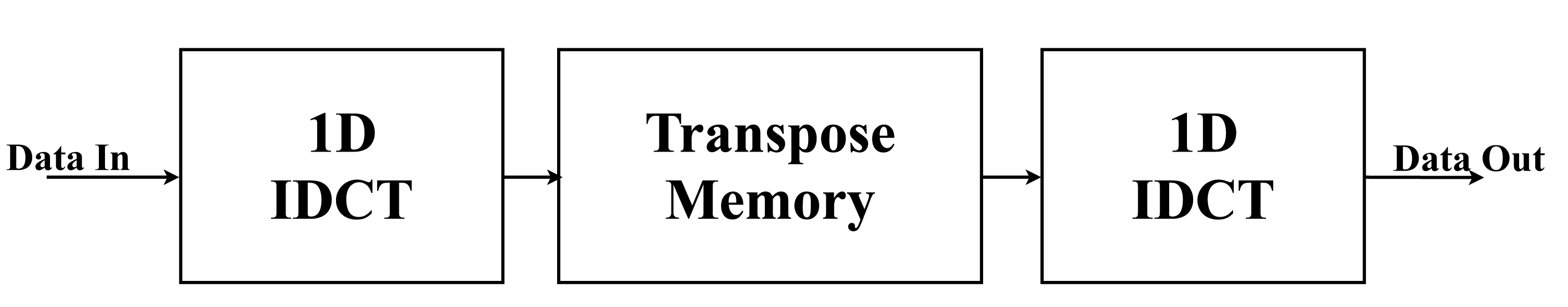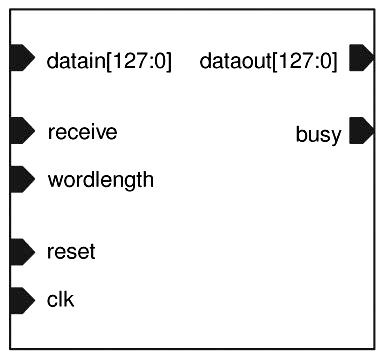## Variable word length processors

Power dissipation is today’s most important problem in digital circuits especially in portable devices. It can be categorized in dynamic and static consumption. Dynamic consumption is generated during CMOS switching while charging and discharging load capacitances. Static consumption is due to subthreshold leakage current. As technology evolves, the latter will grow to become the dominant consumption in integrated circuits.

A great number of studies have focused on reducing a circuit’s power needs either by using advanced hardware or by optimizing the algorithm being implemented. The accuracy of each algorithm is a function of the processor wordlength. Traditionally, processors have used a constant wordlength for each arithmetic operation. Therefore the algorithm’s accuracy is also constant. However, a dynamically varying wordlength can be adjusted depending on the requirements. For a maximum accuracy a larger wordlength is used. Likewise, for power saving a smaller wordlength is used. This technique is independent of the implementation technology. If it is coimplemented with the above methods, further power reduction can be accomplished.

The requirements that were mentioned are frequently found in digital signal processing applications, such as image compression. For example, a video being displayed in a browser window needs less accuracy than when it is displayed in full-screen, consequently a smaller wordlength can be used in order to save power. The first stage of an image compression sequence is the transform of blocks of picture samples into spatial frequency components. Usually the Discrete Cosine Transform (DCT) is selected for this process. During the image decompression, the Inverse Discrete Cosine Transform (IDCT) is used. However, both transforms are compute intensive and need a significant amount of processing power. For example, the percentage of power consumed during the H.263+ video decoding is 21%. Similarly for an MPEG-2 video it is 25%. As a result, the use of a variable processor wordlength can reduce this consumption.

THE INVERSE DCT ALGORITHM

The DCT was proposed by Ahmed et al. in 1974 and is used to transform a spatial waveform into its frequency components. It is the basis of the majority of image compression algorithms because the human eye is less sensitive to higher frequencies in small image blocks. Thus, by using a variable quantization step for each coefficient, data in the higher frequencies can easily be discarded, reducing the file size.

Due to its great hardware complexity, O(N4), various algorithms for the fast computation have been proposed such as Chen’s and Loeffler’s. These are one dimensional algorithms, but a 2-D DCT and IDCT can be obtained by applying these algorithms firstly to every row and secondly to every column. The Loeffler’s algorithm needs 14 multiplications for the computation of the IDCT of each dimension, thus 224 multiplications for an 8x8 block (used in many image compression protocols). This is just 5% of the 4096 multiplications needed by computing it analytically, using the IDCT definition. The accuracy of the inverse DCT is studied, as it is used in image and video decoders which are more frequently found in portable devices.VARIABLE WORDLENGTH EMULATION

The next step is to study the relation between the algorithm’s accuracy and the processor’s wordlength. Since it is commonly used in image processing, the peak signal to noise ratio (PSNR) is selected in order to measure the mean squared error between two images. It is defined as (3) where x is the number of rows, y the number of columns, Y1 and Y2 the luminance value of each image and R is the maximum fluctuation in the input image data type.

The Loeffler’s algorithm is inserted into Matlab’s Simulink and the PSNR is measured between the fast and the analytical approach. As input data, MPEG-4 part 2 test video sequences are used (akiyo, coastguard, container, foreman, hall, mother, news and silent).For this design, a fixed-point number representation is used with a QN-1 number format, where N is the processor’s wordlength. The first bit of the number determines its sign (0: positive, 1: negative) and the rest its magnitude. If the number is negative, a 2‘s complement format is used. As the magnitude of each coefficient is less than 1, each number will have a minimum difference of 1/2N-1. To implement this property in the algorithm a series of quantizers are added after each operation. By changing the quantization interval, the operation will be calculated with a different wordlength. Furthermore, the wordlength can be determined globally, or a different can be used for each stage, group of operations etc.A global wordlength is used for all the operations. PSNR measurements are taken from a 2-bit processor up to 64-bit (Simulink’s maximum). The following figure shows the average PSNR of all the video sequences for each wordlength. It can be seen that the typical SNR rule is generally obeyed, each extra quantization bit increases the dynamic range by 6dB.

PROCESSOR ARCHITECTURE AND PEROFRMANCE

To implement the variable wordlength architecture, a processor is designed written in VHDL. The following figure shows the inputs and outputs of the processor. Its core is the IDCT computation block which contains 13 adders, 13 subtracters and 14 multipliers needed for a 1-D transform. Since the IDCT precision standard (ISO/IEC 230002-1, replacement of former IEEE 1180 specification) demands a minimum 12-bit wordlength, the processor is designed with two available global wordlengths, 12-bit and 16-bit. Input data come in groups of 8 coefficients each clock cycle, thus 8 cycles are needed to input all the coefficients. The data are then computed for each column and the intermediate results are saved in the memory. When the computation for all the columns is finished, the data are transposed, fed again to IDCT block to be computed for each row and sent to the output in groups of 8. The input “wordlength” determines the accuracy of all the arithmetic operations. The IDCT block contains 75% of the processor’s gates so a decrease in power dissipation of the block will significantly decrease the processor’s overall consumption.For the addition operation, two ripple-carry adders were used, connected in series, one 12-bit and one 4-bit. In 12-bit mode, only the first adder is active, while on 16-bit mode, both adders are used with the output carry of the first adder being fed to the input carry of the second one. What is more, in order to support different wordlength for each operation, for future work, three selectors are connected, one for each operand and one for the result. Their task is to ensure that each number stays in the correct format in order to be used by other operations with different wordlengths. In this example, the input selectors feed either all the bits, or the 12 most significant bits to the operator and the result selector outputs all the bits, or the result of the 12-bit adder adjoined with 0’s for the 4 least significant bits.

The structure of the variable length subtractor is designed using the same approach as that of the adder. The implementation of a subtraction using an adder is very common. The first operand is input as is, while the second in 2’s complement format. Therefore, the operand is inverted (1’s complement) and the adder has an input carry 1. There are two selectors in the inputs and one the outputs as before.

An array multiplier is used for the multiplications. The signed multiplication demands that each product of an AND gate which contains one sign bit is inverted and that a constant 2N is added. These properties are implemented using multiplexers. Unlike the adder and the subtracter, the multiplier’s product has twice the length of an operand. The result must be shifted right in order to stay on the Q number format. As with the other operations, two selectors in the inputs and one in the output exist.

The complete architecture is synthesized into a Xilinx Virtex 5 FPGA and is compared to a 16-bit constant wordlength processor with the same architecture. When an adder is used in 12-bit mode it consumes 97% of the dynamic power of a 16-bit constant wordlength adder. When used in 16-bit mode it reaches 124%. This is due to the addition of the three selectors in order for the adder to be independent of the wordlength used in the other operators. The selectors double the amount of gates needed and diminish the energy dissipation gain when used in 12-bit mode. The overhead of the selectors in the multiplier is smaller due to its complexity. Consequently, the increase in number of gates is just 10%. When in 12-mode the multiplier consumes 78% of the power of a 16-bit constant wordlength multiplier with the same architecture and 118% when in 16-bit mode. By combining the results of all the operators, the IDCT block needs 90% when in 12-bit mode and 122% when in 16-bit mode. Consequently, if the 12-bit mode is needed more frequently than the 16-bit mode, the processor will dissipate less power.

In FPGA technology, only a diminish in dynamic power consumption can be achieved. However, having discretized the units needed for 12-bit and 16-bit operations, in ASIC technology power supply can be cut to the units not needed which will lead to a decrease in static power consumption and a power dissipation overall.